# How many lumber in a cube: the volume of timber (calculation table)

The volume of a unit of cut timber having standard dimensions is easily determined. Therefore, having a project at home, it is easy to calculate how much a bar (in pieces) will be required for the construction, for example, of its walls. When calculating should take into account the size of window openings and other places where wood replaces other materials.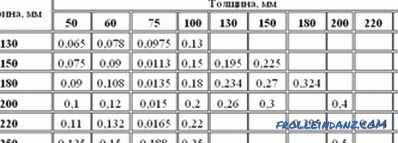Table of calculation of the cubic capacity of the log and timber.

In determining the required volume, it is necessary to take into account such a property of wood as shrinkage. If the tree dries before stacking into the walls while stacked on the construction site, a decrease in moisture below 25‑30% will quite significantly affect its transverse dimensions: shrinkage of transverse dimensions can be 6‑9%. Volume shrinkage reaches 12‑15%.

## Calculation of the required volume of timber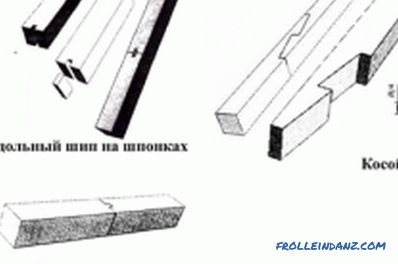Figure 1. Methods of longitudinal connection with the remainder.

The thickness of the walls of a wooden private house, excluding insulation, is usually 200 mm. That is, a standard timber is suitable, in the cross section of which one of the sides is 20 cm. For example, 150 * 200 (option 1) or 200 * 200 (option 2) with a length of 6 m. For areas with a more severe climate, you may need a section 150 * 300 (option 3).

The walls of the house can have a width or length exceeding 6 m. Different types of locks are used for the longitudinal connection in the crown: a straight or oblique padlock. Each such connection reduces the length of the connected parts, which must be compensated. The same applies to the corner joint, if it is performed with the remainder.

Study the methods of longitudinal connection (image 1) and the method of angular connection with the rest (image 2).

The number of crowns depends on the height of the structure and on the cross-section of the timber.

Let the planned height of the room is 2.6 m. The number of crowns in option 1 and 3 is: 2.6: 0.15 = 17.33 pcs. Between the crowns there will be 16 gaps, which will be filled with insulation 5 mm thick. The total thickness of the insulation is 80 mm. Therefore, the height of the room is equal to: 17 (crowns) * 150 (thickness) + 16 (gaps) * 5 = 2630 (mm).

It is necessary to add another crown - the basement. For the exterior walls of a single-storey building, 18 crowns are required.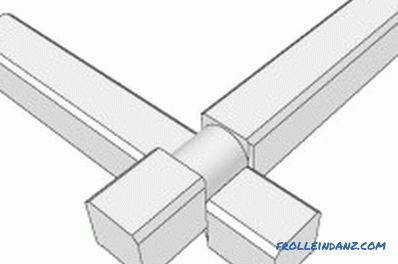Figure 2. The method of the corner connection with the remainder.

It is necessary to perform the same calculation for option 2:

• number of crowns: 2.6: 0.2 = 13;
• room height: 13 * 200 +12 * 5 = 2660 (mm).

The total number of crowns (including the basement) is 14 pcs.

The change in cross section influenced the height of the room, which will affect the volume of lumber purchased. Since the total length has not changed, it is sufficient to compare only the cross-sectional area of ​​the walls. Thus, the areas are 15 * 20 * 18 = 5400 cm², 20 * 20 * 14 = 5600 cm² and 15 * 30 * 18 = 8100 cm². Regarding option 1, the increase was 4% and 50%. The difference in cost may be even greater.

It can be assumed: the size of the building is chosen so that only one beam is enough for the width, and two are enough for the length (taking into account the longitudinal connection), that is, 6 units will be used for one crown.

Depending on the variant, 108 pieces will be required. 84 pcs. and 108 pcs.

### The amount of material in one cube

If you know how many units and what material is needed for construction, you can determine how many cubes you need.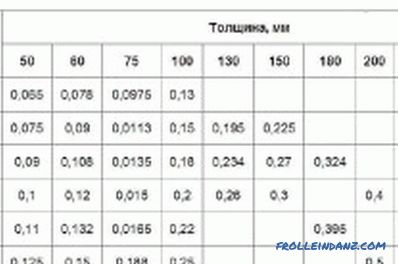Auxiliary table for calculating the cubic capacity of a beam for building a house.

So, depending on the option, you need to purchase:

• V 1 = 0.15 * 0.2 * 6 * 108 = 19, 44 m³;
• V 2 = 0.2 * 0.2 * 6 * 84 = 20.16 m³;
• V 3 = 0.15 * 0.3 * 6 * 108 = 29.16 m³ .

If the seller agrees to sell the goods by the piece, then there will be no problems: multiply the resulting volume by the cost of 1 cube.

In the first version, up to 20 cubes is enough to add 3 bars, and then the total volume will be 19.98 m 3 . Since in a household buying can always be useful, such conditions of purchase are also acceptable.

However, it is not always possible to purchase as many as you wish. For example, the volume of one copy is 0.2 * 0.2 * 6 = 0.24 m³. Therefore, 1 cube contains 1: 0.24 = 4.17 pcs. and the seller logically believes that 4 units occupy a volume of 1 m³. That is, the actual volume was 0.96 m³, and the deficiency is 0.04 m³.

Since 84 such bars are required, or 21 cubic meters (from the seller's point of view), the buyer will actually purchase 0.04 * 21 = 0.84 m³ less, and this is already more than 3 units.

If, according to the terms of the store, you purchased material in option 1, then your losses would be even greater. Indeed, in one cube there should be 1: 0.18 = 5.56 pcs. The drawback on each cube will be: 1-0.18 * 5 = 0.1 m³, that is, instead of 20 cubes, the buyer must buy 22 cubes.

Column 2 of the table indicates consumer losses due to the fact that the sale does not include the fractional part.Losses only on one cube are indicated in column 3 of the table. They are especially large for a section of 0.15 * 0.3. By agreeing to purchase 29 m³ on the terms of the seller, you lose 0.19 * 29 = 5.51 m³ of material.

 Cross-section, m The number of bars with a length of 6 m in one cube Buyer losses when the seller only considers the whole part, m³ 1 2 3 0.1 * 0.2 8.33 0.04 0.15 * 0.2 5.56 0, 1 0.2 * 0.2 4.17 0.04 0.15 * 0.3 3.70 0.19

The table shows the loss of the buyer for only one cube purchased tree. In order to determine the total loss, it is sufficient to multiply the values ​​given in column 3 by the number of cubes purchased.

Therefore, sometimes it makes sense to bargain and prove how unprofitable it is to purchase goods on the proposed terms.

What conclusion follows from the above calculations? You must be ready to defend their interests. For this, the buyer should know what quantity of material of standard sizes is contained in 1 m³, and if the seller takes into account only the whole component of this number, show him how much he is trying to deceive the buyer.

It should be noted that the same problem exists with the purchase of edged boards.

•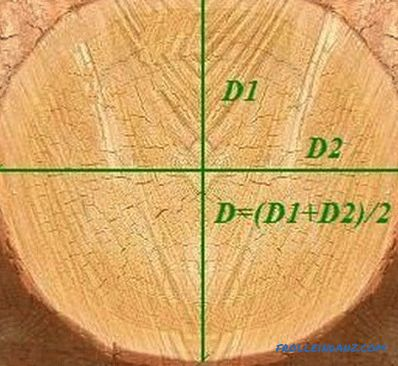How to calculate the log volume correctly?

How to calculate the volume of a log correctly, which calculation methods allow you to accurately estimate the volume of roundwood? Calculation of the volume of occupied space, individual logs, tables.

•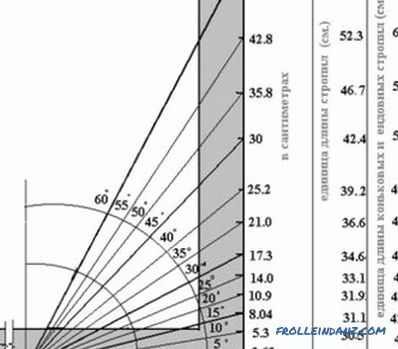How to calculate the length of rafters correctly?

Recommendations on how to calculate the length of the rafters, taking into account the shape of the roof, wind and snow loads. An example of calculating the cross section of a timber and its length. Possible errors in the work.